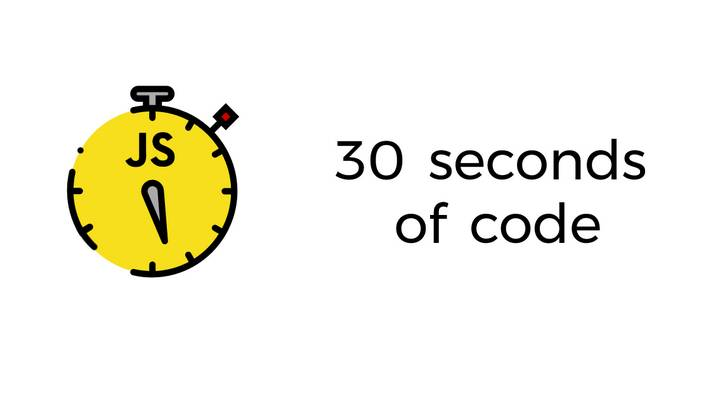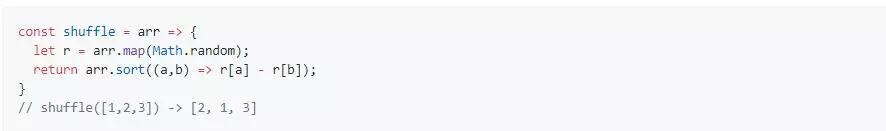# 征服 JavaScript 面试：什么是函数组合

2017/01/30 · JavaScript · 2 评论 · 函数Google 数据中心管道 — Jorge Jorquera — (CC-BY-NC-ND-2.0)

“征服 JavaScript 面试”是我写的一系列文章，来帮助面试者准备他们在面试 JavaScript 中、高级职位中将可能会遇到的一些问题。这些问题我自己在面试中也经常会问。

1. `x`
2. `g`
3. `f`

1. 将姓名根据空格分拆（split）到一个数组中
2. 将姓名映射（map）为小写
3. 用破折号连接（join）
4. 编码 URI 组件

JavaScript

const toSlug = input => encodeURIComponent( input.split(' ') .map(str => str.toLowerCase()) .join('-') );

 1 2 3 4 5 const toSlug = input => encodeURIComponent(   input.split(' ')     .map(str => str.toLowerCase())     .join('-') );

JavaScript

const toSlug = input => encodeURIComponent( join('-')( map(toLowerCase)( split(' ')( input ) ) ) ); console.log(toSlug('JS Cheerleader')); // 'js-cheerleader'

 1 2 3 4 5 6 7 8 9 10 11 const toSlug = input => encodeURIComponent(   join('-')(     map(toLowerCase)(       split(' ')(         input       )     )   ) );   console.log(toSlug('JS Cheerleader')); // 'js-cheerleader'

JavaScript

const curry = fn => (...args) => fn.bind(null, ...args); const map = curry((fn, arr) => arr.map(fn)); const join = curry((str, arr) => arr.join(str)); const toLowerCase = str => str.toLowerCase(); const split = curry((splitOn, str) => str.split(splitOn));

 1 2 3 4 5 6 7 8 9 const curry = fn => (...args) => fn.bind(null, ...args);   const map = curry((fn, arr) => arr.map(fn));   const join = curry((str, arr) => arr.join(str));   const toLowerCase = str => str.toLowerCase();   const split = curry((splitOn, str) => str.split(splitOn));

JavaScript

import { curry, map, join, split } from 'lodash/fp';

 1 import { curry, map, join, split } from 'lodash/fp';

JavaScript

const curry = require('lodash/fp/curry'); const map = require('lodash/fp/map'); //...

 1 2 3 const curry = require('lodash/fp/curry'); const map = require('lodash/fp/map'); //...

JavaScript

const toSlug = input => encodeURIComponent( join('-')( map(toLowerCase)( split(' ')( input ) ) ) ); console.log(toSlug('JS Cheerleader')); // 'js-cheerleader'

 1 2 3 4 5 6 7 8 9 10 11 const toSlug = input => encodeURIComponent(   join('-')(     map(toLowerCase)(       split(' ')(         input       )     )   ) );   console.log(toSlug('JS Cheerleader')); // 'js-cheerleader'

JavaScript

const compose = (...fns) => x => fns.reduceRight((v, f) => f(v), x);

 1 const compose = (...fns) => x => fns.reduceRight((v, f) => f(v), x);

`.reduce()` 一样，数组的 `.reduceRight()` 方法带有一个 reducer 函数和一个初始值（`x`）为参数。我们可以用它从右到左迭代数组，将函数依次应用到每个数组元素上，最后得到累加值（`v`）。

`compose`，我们就可以不需要嵌套来重写上面的组合：

JavaScript

const toSlug = compose( encodeURIComponent, join('-'), map(toLowerCase), split(' ') ); console.log(toSlug('JS Cheerleader')); // 'js-cheerleader'

 1 2 3 4 5 6 7 8 const toSlug = compose(   encodeURIComponent,   join('-'),   map(toLowerCase),   split(' ') );   console.log(toSlug('JS Cheerleader')); // 'js-cheerleader'

JavaScript

import { compose } from 'lodash/fp';

 1 import { compose } from 'lodash/fp';

JavaScript

const compose = require('lodash/fp/compose');

 1 const compose = require('lodash/fp/compose');

JavaScript

const pipe = (...fns) => x => fns.reduce((v, f) => f(v), x); const fn1 = s => s.toLowerCase(); const fn2 = s => s.split('').reverse().join(''); const fn3 = s => s + '!' const newFunc = pipe(fn1, fn2, fn3); const result = newFunc('Time'); // emit!

 1 2 3 4 5 6 7 8 const pipe = (...fns) => x => fns.reduce((v, f) => f(v), x);   const fn1 = s => s.toLowerCase(); const fn2 = s => s.split('').reverse().join(''); const fn3 = s => s + '!'   const newFunc = pipe(fn1, fn2, fn3); const result = newFunc('Time'); // emit!

JavaScript

const toSlug = pipe( split(' '), map(toLowerCase), join('-'), encodeURIComponent ); console.log(toSlug('JS Cheerleader')); // 'js-cheerleader'

 1 2 3 4 5 6 7 8 const toSlug = pipe(   split(' '),   map(toLowerCase),   join('-'),   encodeURIComponent );   console.log(toSlug('JS Cheerleader')); // 'js-cheerleader'

JavaScript

const trace = curry((label, x) => { console.log(`== \${ label }: \${ x }`); return x; });

 1 2 3 4 const trace = curry((label, x) => {   console.log(`== \${ label }:  \${ x }`);   return x; });

JavaScript

const toSlug = pipe( trace('input'), split(' '), map(toLowerCase), trace('after map'), join('-'), encodeURIComponent ); console.log(toSlug('JS Cheerleader')); // '== input: JS Cheerleader' // '== after map: js,cheerleader' // 'js-cheerleader'

 1 2 3 4 5 6 7 8 9 10 11 12 13 const toSlug = pipe(   trace('input'),   split(' '),   map(toLowerCase),   trace('after map'),   join('-'),   encodeURIComponent );   console.log(toSlug('JS Cheerleader')); // '== input:  JS Cheerleader' // '== after map:  js,cheerleader' // 'js-cheerleader'

`trace()` 只是更通用的 `tap()` 的一种特殊形式，它可以让你对流过管道的每个值执行一些行为。明白了么？管道（Pipe）？水龙头(Tap)？可以像下面这样编写 `tap()`

JavaScript

const tap = curry((fn, x) => { fn(x); return x; });

 1 2 3 4 const tap = curry((fn, x) => {   fn(x);   return x; });

JavaScript

const trace = label => { return tap(x => console.log(`== \${ label }: \${ x }`)); };

 1 2 3 const trace = label => {   return tap(x => console.log(`== \${ label }:  \${ x }`)); };

1 赞 9 收藏 2 评论Anagrams of string（带有重复项）

const anagrams = str => {

if (str.length <= 2) return str.length === 2 ? [str, str + str] : [str];

return str.split('').reduce((acc, letter, i) =>

acc.concat(anagrams(str.slice(0, i) + str.slice(i + 1)).map(val => letter + val)), []);

};

// anagrams('abc') -> ['abc','acb','bac','bca','cab','cba']

const average = arr => arr.reduce((acc, val) => acc + val, 0) / arr.length;

// average([1,2,3]) -> 2

const capitalizeEveryWord = str => str.replace(/[a-z]/g, char => char.toUpperCase());

// capitalizeEveryWord('hello world!') -> 'Hello World!'

const capitalize = (str, lowerRest = false) =>

str.slice(0, 1).toUpperCase() + (lowerRest ? str.slice(1).toLowerCase() : str.slice(1));

// capitalize('myName', true) -> 'Myname'

const palindrome = str => {

const s = str.toLowerCase().replace(/[W_]/g,'');

return s === s.split('').reverse().join('');

}

// palindrome('taco cat') -> true

const countOccurrences = (arr, value) => arr.reduce((a, v) => v === value ? a + 1 : a + 0, 0);

// countOccurrences([1,1,2,1,2,3], 1) -> 3

const currentUrl = _ => window.location.href;

// currentUrl() -> ''

Curry

const curry = (fn, arity = fn.length, ...args) =>

arity <= args.length

? fn(...args)

: curry.bind(null, fn, arity, ...args);

// curry(Math.pow)(2)(10) -> 1024

// curry(Math.min, 3)(10)(50)(2) -> 2

Deep flatten array

const deepFlatten = arr =>

arr.reduce((a, v) => a.concat(Array.isArray(v) ? deepFlatten(v) : v), []);

// deepFlatten([1,,[,4],5]) -> [1,2,3,4,5]

const difference = (a, b) => { const s = new Set(b); return a.filter(x => !s.has(x)); };

// difference([1,2,3], [1,2]) -> 

const distance = (x0, y0, x1, y1) => Math.hypot(x1 - x0, y1 - y0);

// distance(1,1, 2,3) -> 2.23606797749979

const isDivisible = (dividend, divisor) => dividend % divisor === 0;

// isDivisible(6,3) -> true

const escapeRegExp = str => str.replace(/[.*+?^\${}()|[]]/g, '\$&');

// escapeRegExp('(test)') -> (test)

const isEven = num => num % 2 === 0;

// isEven(3) -> false

const factorial = n => n <= 1 ? 1 : n * factorial(n - 1);

// factorial(6) -> 720

const fibonacci = n =>

Array(n).fill(0).reduce((acc, val, i) => acc.concat(i > 1 ? acc[i - 1] + acc[i - 2] : i), []);

// fibonacci(5) -> [0,1,1,2,3]

const filterNonUnique = arr => arr.filter(i => arr.indexOf(i) === arr.lastIndexOf(i));

// filterNonUnique([1,2,2,3,4,4,5]) -> [1,3,5]

Flatten数组

const flatten = arr => arr.reduce((a, v) => a.concat(v), []);

// flatten([1,,3,4]) -> [1,2,3,4]

const arrayMax = arr => Math.max(...arr);

// arrayMax([10, 1, 5]) -> 10

const arrayMin = arr => Math.min(...arr);

// arrayMin([10, 1, 5]) -> 1

const getScrollPos = (el = window) =>

({x: (el.pageXOffset !== undefined) ? el.pageXOffset : el.scrollLeft,

y: (el.pageYOffset !== undefined) ? el.pageYOffset : el.scrollTop});

// getScrollPos() -> {x: 0, y: 200}

const gcd = (x, y) => !y ? x : gcd(y, x % y);

// gcd (8, 36) -> 4

const head = arr => arr;

list初始化

const initial = arr => arr.slice(0, -1);

// initial([1,2,3]) -> [1,2]

const initializeArrayRange = (end, start = 0) =>

Array.apply(null, Array(end - start)).map((v, i) => i + start);

// initializeArrayRange(5) -> [0,1,2,3,4]

const initializeArray = (n, value = 0) => Array(n).fill(value);

// initializeArray(5, 2) -> [2,2,2,2,2]

const last = arr => arr.slice(-1);

// last([1,2,3]) -> 3

const timeTaken = callback => {

console.time('timeTaken');

const r = callback();

console.timeEnd('timeTaken');

return r;

};

// timeTaken(() => Math.pow(2, 10)) -> 1024

// (logged): timeTaken: 0.02099609375ms

const objectFromPairs = arr => arr.reduce((a, v) => (a[v] = v, a), {});

// objectFromPairs([['a',1],['b',2]]) -> {a: 1, b: 2}

const pipe = (...funcs) => arg => funcs.reduce((acc, func) => func(acc), arg);

// pipe(btoa, x => x.toUpperCase())("Test") -> "VGVZDA=="

Powerset

const powerset = arr =>

arr.reduce((a, v) => a.concat(a.map(r => [v].concat(r))), [[]]);

// powerset([1,2]) -> [[], , , [2,1]]

const randomIntegerInRange = (min, max) => Math.floor(Math.random() * (max - min + 1)) + min;

// randomIntegerInRange(0, 5) -> 2

const randomInRange = (min, max) => Math.random() * (max - min) + min;

// randomInRange(2,10) -> 6.0211363285087005

const shuffle = arr => arr.sort(() => Math.random() - 0.5);

// shuffle([1,2,3]) -> [2,3,1]

const redirect = (url, asLink = true) =>

asLink ? window.location.href = url : window.location.replace(url);

// redirect('')

const reverseString = str => [...str].reverse().join('');

// reverseString('foobar') -> 'raboof'

RGB到十六进制

const rgbToHex = (r, g, b) => ((r << 16) + (g << 8) + b).toString(16).padStart(6, '0');

// rgbToHex(255, 165, 1) -> 'ffa501'

const scrollToTop = _ => {

const c = document.documentElement.scrollTop || document.body.scrollTop;

if (c > 0) {

window.requestAnimationFrame(scrollToTop);

window.scrollTo(0, c - c / 8);

}

};

// scrollToTop()const similarity = (arr, values) => arr.filter(v => values.includes(v));

// similarity([1,2,3], [1,2,4]) -> [1,2]

const sortCharactersInString = str =>

str.split('').sort((a, b) => a.localeCompare(b)).join('');

// sortCharactersInString('cabbage') -> 'aabbceg'

const sum = arr => arr.reduce((acc, val) => acc + val, 0);

// sum([1,2,3,4]) -> 10

[varA, varB] = [varB, varA];

// [x, y] = [y, x]

const tail = arr => arr.length > 1 ? arr.slice(1) : arr;

// tail([1,2,3]) -> [2,3]

// tail() -> 

const unique = arr => [...new Set(arr)];

// unique([1,2,2,3,4,4,5]) -> [1,2,3,4,5]

URL参数

const getUrlParameters = url =>

url.match(/([^?=&]+)(=([^&]*))/g).reduce(

(a, v) => (a[v.slice(0, v.indexOf('='))] = v.slice(v.indexOf('=') + 1), a), {}

);

// getUrlParameters('') -> {name: 'Adam', surname: 'Smith'}

UUID生成器

const uuid = _ =>

([1e7] + -1e3 + -4e3 + -8e3 + -1e11).replace(//g, c =>

(c ^ crypto.getRandomValues(new Uint8Array(1)) & 15 >> c / 4).toString(16)

);

// uuid() -> '7982fcfe-5721-4632-bede-6000885be57d'

const validateNumber = n => !isNaN(parseFloat(n)) && isFinite(n) && Number(n) == n;

// validateNumber('10') -> true